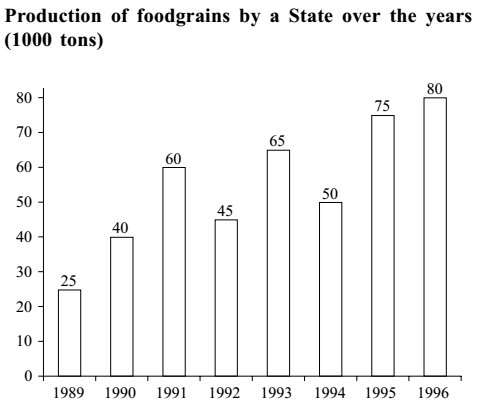#### Data Interpretation

Direction: Study the following graph carefully and answer the questions given below it.1. In how many of the given years was the production of foodgrains more than average production of the given years?
1. 2
2. 3
3. 4
4. 1
5. None of these
##### Correct Option: C

Average production

 = 15 + 40 + 60 + 45 + 65 + 50 + 70 + 80 8

 = 440 = 55 . 8

The average production of the year is 55 and as we are seeing in the graph that the year 1991, 1993, 1995, 1996 have more than the 55.